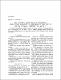## The p-groups satisfying the condition: each cyclic subgroup is contained in the center or has a trivial intersection with it2006
Pylyavska, Olha
Shatohina, Ju.
##### Abstract
Y. Berkovich proposed the next problem: "Suppose that p-group G satisfies the following condition: if Z is a cyclic subgroup o f G then either Z ≤ Z(G) or Z ⋂ Z(G) = {1}. Classify all such groups". We have proved that abelian p-groups and p-groups with exponent p exhaust all regular p-groups satisfying this condition.
##### Keywords
Р-групи, тривіальний перетин, З.Янк, В.Чепулічу, циклічна підгрупа, p-group
##### Citation
Pylyavska Olha, The p-groups satisfying the condition : each cyclic subgroup is contained in the center or has a trivial intersection with it / O. Pylyavska, Ju. Shatohina // Наукові записки НаУКМА. - 2006. - Т. 51 : Фізико-математичні науки. - С. 3-4.